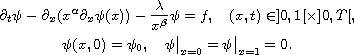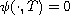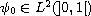Electron. J. Differential Equations, Vol. 2017 (2017), No. 135, pp. 1-17.

### Data assimilation and null controllability of degenerate/singular parabolic problems Khalid Atifi, El-Hassan Essoufi

Abstract:
In this article, we use the variational method in data assimilation to study numerically the null controllability of degenerate/singular parabolic problemTo do this, we determine the source term f with the aim of obtaining, for all. This problem can be formulated in a least-squares framework, which leads to a non-convex minimization problem that is solved using a regularization approach. Also we present some numerical experiments.

Submitted February 24, 2017. Published May 17, 2017.
Math Subject Classifications: 15A29, 47A52, 93C20, 35K05, 35K65, 35K65, 93B05.
Key Words: Data assimilation; null controllability; regularization; heat equation; inverse problem; degenerate equations; optimization.

Show me the PDF file (331 KB), TEX file for this article.Khalid Atifi Laboratoire de Mathématiques Informatique et Sciences de l'ingénieur (MISI) Université Hassan 1 Settat 26000, Morocco email: k.atifi.uhp@gmail.com El-Hassan Essoufi Laboratoire de Mathématiques Informatique et Sciences de l'ingénieur (MISI) Université Hassan 1 Settat 26000, Morocco email: e.h.essoufi@gmail.com